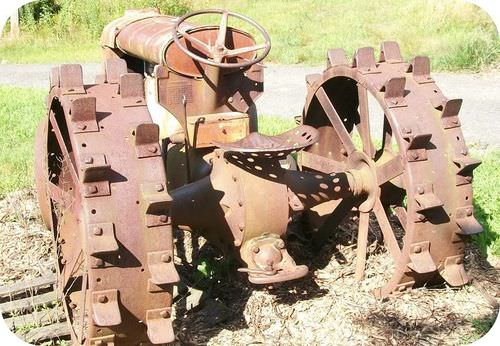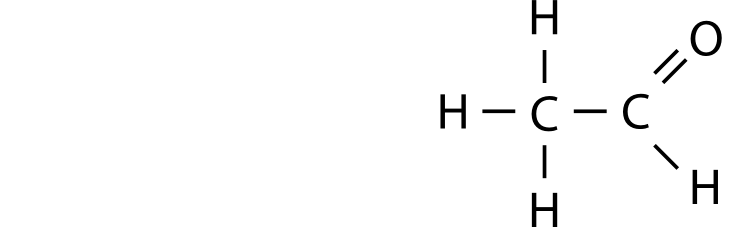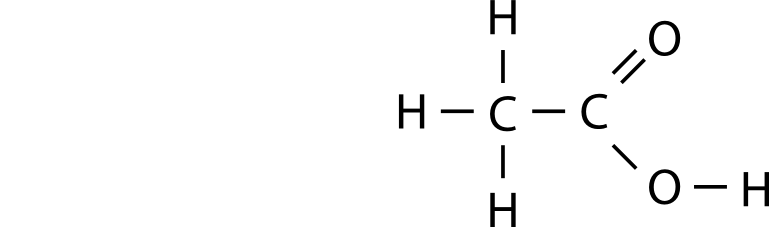# 11.2: The Nature of Oxidation and Reduction

•• Contributed by Allison Soult
• Senior Lecturer (Chemistry) at University of Kentucky

Learning Outcomes

• Define oxidation and reduction in terms of a gain or loss of oxygen or hydrogen atoms.
• Define oxidation and reduction in terms of a gain or loss of electrons.
• Identify the substances involved in oxidation and reduction in a reaction.
• Identify the oxidizing and reducing agents in a redox reaction.
• Write the oxidation or reduction sequence for a molecule (alcohol, aldehyde/ketone, carboxylic acid)

Oxygen is an element that has been known for centuries. In its pure elemental form, oxygen is highly reactive, and it readily makes compounds with most other elements. It is also the most abundant element by mass in the Earth's crust. The class of reactions called oxidation and reduction were originally defined with respect to the element oxygen.

## Oxygen's Role in Reactions

Many elements simply combine with oxygen to form the oxide of that element. Heating magnesium in air allows it to combine with oxygen to form magnesium oxide.

$2 \ce{Mg} \left( s \right) + \ce{O_2} \left( g \right) \rightarrow 2 \ce{MgO} \left( s \right)$

Many compounds react with oxygen as well, often in very exothermic processes that are generally referred to as combustion reactions. For example, when methane burns, carbon dioxide and water are produced.

$\ce{CH_4} \left( g \right) + 2 \ce{O_2} \left( g \right) \rightarrow \ce{CO_2} \left( g \right) + 2 \ce{H_2O} \left( g \right)$

Carbon dioxide is an oxide of carbon, while water is an oxide of hydrogen. Early scientists viewed oxidation as a process in which a substance was reacted with oxygen to produce one or more oxides. In the previous examples, magnesium and methane are being oxidized.

Oxidation does not necessarily require heating. Iron that is exposed to air and water slowly oxidizes in a process commonly known as rusting. Bleaches contain various compounds such as sodium hypochlorite $$\left( \ce{NaClO} \right)$$ that can oxidize stains by the transfer of oxygen atoms, making the molecules in the stains more water-soluble and therefore easier to rinse off. Hydrogen peroxide $$\left( \ce{H_2O_2} \right)$$ releases oxygen as it spontaneously decomposes. It also acts as a bleach and is ued as an antiseptic that kills bacteria by oxidizing them.

The opposite of oxidation is called reduction. Since oxidation was originally defined as the addition of oxygen, reduction was therefore the removal of oxygen from a substance. Many naturally occurring metal ores are present as oxides. The pure metals can be extracted by reduction. For example, pure iron is obtained from iron (III) oxide by reacting it with carbon at high temperatures.

$2 \ce{Fe_2O_3} \left( s \right) + 3 \ce{C} \left( s \right) \rightarrow 4 \ce{Fe} \left( s \right) + 3 \ce{CO_2} \left( g \right)$

The removal of oxygen from the $$\ce{Fe_2O_3}$$ molecule means that it is being reduced to $$\ce{Fe}$$. Note that an oxidation process is also occurring simultaneously in this reaction; the carbon reactant is being oxidized to $$\ce{CO_2}$$. This is an important concept. In looking at organic compounds, we can describe oxidation and reduction in terms of the loss or gain of oxygen or the loss or gain of hydrogen.

If we look at the oxidation of ethanol ($$\ce{CH_3CH_2OH}$$, an alcohol) to form ethanal ($$\ce{CH_3CH_2CHO}$$, an aldehyde), we see that the number of bonds to oxygen has increased and the number of hydrogen atoms has decreased from six to four. Either or both of these indicate that an oxidation has occurred.For a reduction, we look for the removal of oxygen or bonds to oxygen or the addition or hydrogen atoms. In the following reaction, we see ethanoic acid ($$\ce{CH_3COOH}$$, a carboxylic acid) being reduced to ethanal ($$\ce{CH_3CH_2CHO}$$, an aldehyde). Note that the number of oxygen atoms is reduced from two to one. There is no change in the number of hydrogen atoms, but both changes do not need to happen for evidence of oxidation or reduction.If we see evidence of an oxidation or reduction, we know the other must have happened as well. Oxidation and reduction must happen together. Neither can happen alone in a reaction.

### The Electron in Redox Reactions

The definitions of oxidation and reduction were eventually broadened to include similar types of reactions that do not necessarily involve oxygen. Oxygen is more electronegative than any element except for fluorine. Therefore, when oxygen is bonded to any element other than fluorine, electrons from the other atom are shifted away from that atom and toward the oxygen atom. An oxidation-reduction reaction (sometimes abbreviated as a redox reaction) is a reaction that involves the full or partial transfer of electrons from one reactant to another. Oxidation involves a full or partial loss of electrons, while reduction involves a full or partial gain of electrons.

An easy saying to remember the definitions of oxidation and reduction is "LEO the lion says GER" (see figure below)! LEO stands for Losing Electrons is Oxidation, while GER stands for Gaining Electrons is Reduction.Figure $$\PageIndex{1}$$: This guy knows his redox reactions.

### Redox Reactions and Ionic Compounds

In the course of a chemical reaction between a metal and a nonmetal, electrons are transferred from the metal atoms to the nonmetal atoms. For example, when zinc metal is heated in the presence of sulfur, the compound zinc sulfide is produced (see figure below). Two valence electrons from each zinc atom are transferred to each sulfur atom.Since zinc is losing electrons during this reaction, it is being oxidized. The sulfur is gaining electrons, so it is being reduced. Each of these processes can be shown in a separate equation called a half-reaction. A half-reaction is an equation that shows either the oxidation or the reduction reaction that occurs during a redox reaction.

\begin{align} &\text{Oxidation:} \: \: \ce{Zn} \rightarrow \ce{Zn^{2+}} + 2 \ce{e^-} \\ &\text{Reduction:} \: \: \ce{S} + 2 \ce{e^-} \rightarrow \ce{S^{2-}} \end{align}

It is important to remember that the two half-reactions occur simultaneously. The resulting ions that are formed are then attracted to one another, forming an ionic bond.

Redox reactions are also commonly described in terms of oxidizing and reducing agents. In the reaction above, the zinc is being oxidized by losing electrons. However, an isolated ionization would be a very high-energy process, so there must be another substance present to gain those lost electrons. In this case, the electrons are gained by sulfur. In other words, the sulfur is causing the zinc to be oxidized. Consequently, sulfur is referred to as the oxidizing agent. Conversely, the zinc causes the sulfur to gain electrons and become reduced, so zinc is the reducing agent. An oxidizing agent is a substance that causes oxidation by accepting electrons, and a reducing agent is a substance that causes reduction by losing electrons. Said another way, the oxidizing agent is the substance that is reduced, while the reducing agent is the substance that is oxidized.

Example $$\PageIndex{1}$$

When chlorine gas is bubbled into a solution of sodium bromide, a reaction occurs that produces aqueous sodium chloride and elemental bromine.

1. Determine what is being oxidized and what is being reduced.
2. Identify the oxidizing and reducing agents.

Solution

First, look at each element in the reaction and assign oxidation numbers to each element.

$\ce{Cl_2} \left( g \right) + 2 \ce{NaBr} \left( aq \right) \rightarrow 2 \ce{NaCl} \left( aq \right) + \ce{Br_2} \left( l \right)$

Chlorine in $$\ce{Cl_2}$$ and bromine in $$\ce{Br_2}$$ both have oxidation numbers of 0. $$\ce{NaBr}$$ and $$\ce{NaCl}$$ are ionic compounds so their ionic charges will be their oxidation numbers.

$\overset{0}{\ce{Cl_2}} \left( g \right) + 2 \overset{+1}{\ce{Na}} \overset{-1}{\ce{Br}} \left( aq \right) \rightarrow 2 \overset{+1}{\ce{Na}} \overset{-1}{\ce{Cl}} \left( aq \right) + \overset{0}{\ce{Br_2}} \left( l \right)$

a. The oxidation state of chlorine goes from 0 to $$-1$$ so it is gaining an electron and being reduced. The oxidation state of bromine goes from $$-1$$ to 0 so it is losing an electron and being oxidized. The oxidation state of sodium does not change so it is not involved in either the oxidation or the reduction.

b. The $$\ce{Cl_2}$$ is being reduced and is the oxidizing agent. The $$\ce{Br^-}$$ is being oxidized and is the reducing agent.Figure $$\PageIndex{2}$$: Rusting is a form of corrosion, a process in which metals are oxidized.

### Redox Reactions and Molecular Compounds

The loss or gain of electrons is easy to see in a reaction in which ions are formed. However, in many reactions, electrons are not transferred completely. Recall that in a molecular compound, electrons are shared between atoms in a type of bond called a covalent bond. Even though electrons are not completely transferred between atoms, it is still common for reactions involving molecular compounds to be classified as redox reactions.

For example, when hydrogen gas is reacted with oxygen gas, water is formed as the product.

$2 \ce{H_2} \left( g \right) + \ce{O_2} \left( g \right) \rightarrow 2 \ce{H_2O} \left( l \right)$

We assign oxidation numbers to determine which species are gaining and losing electrons. Remember oxidation numbers tell us what the charge would be if the electrons were transferred completely even though they are shared, not transferred, in covalent bonds. For the synthesis of water, the oxidation numbers of the free elements (hydrogen and oxygen) are zero. For water, oxygen has a $$-2$$ oxidation number while hydrogen has a $$+1$$.

$2 \overset{0}{\ce{H_2}} \left( g \right) + \overset{0}{\ce{O_2}} \left( g \right) \rightarrow 2 \overset{+1}{\ce{H_2}} \overset{-2}{\ce{O}} \left( l \right)$

The oxidation state from hydrogen goes from 0 to $$+1$$, indicating oxidation. The oxidation state of oxygen goes from 0 to $$-2$$, indicating reduction. Therefore hydrogen is oxidized and is the reducing agent. Oxygen is reduced and is the oxidizing agent.

#### Gain or Loss of Hydrogen and Oxygen

Oxidation can also be defined as the addition of oxygen to a molecule or the removal of hydrogen. Reduction is therefore the addition of hydrogen or the removal of oxygen. Several classes of organic compounds are related to one another by oxidation and reduction reactions. Alkanes, alkenes, and alkynes represent different levels of oxidation of a hydrocarbon. When an alkane is heated in the presence of an appropriate catalyst, it can be oxidized to the corresponding alkene in a reaction called a dehydrogenation reaction. Two hydrogen atoms are removed in the process. The alkene can be further oxidized to an alkyne by the removal of two more hydrogen atoms. This is also considered an oxidation according to the modern definition, because the oxidation number of each carbon atom goes from $$-3$$ to $$-2$$ to $$-1$$.

$\text{oxidation:} \: \: \ce{CH_3CH_3} \overset{\ce{-H_2}}{\rightarrow} \ce{CH_2=CH_2} \overset{\ce{-H_2}}{\rightarrow} \ce{CH \equiv CH}$

The reactions are reversible, so an alkyne can be reduced first to an alkene and then to an alkane. These are addition reactions, as seen in the previous section.

$\text{reduction:} \: \: \ce{CH \equiv CH} \overset{\ce{+H_2}}{\rightarrow} \ce{CH_2=CH_2} \overset{\ce{+H_2}}{\rightarrow} \ce{CH_3CH_3}$

The alkane is the most reduced form of a hydrocarbon, while the alkyne is the most oxidized form.

The changes in electrons, oxidation number, number of oxygen atoms, and number of hydrogen atoms is summarized below for oxidation and reduction reactions.

Oxidation reactions in organic chemistry often involve the addition of oxygen to a compound (or an increase in the number of bonds to oxygen), which changes the functional group that is present. The following sequence shows how methane can be oxidized first to methanol (alcohol), then to methanal (aldehyde), then to methanoic acid (carboxylic acid), and finally to carbon dioxide. Each step in the process is either a gain of oxygen or a loss of hydrogen. Each step also represents energy, which explains why the complete combustion of alkanes to carbon dioxide is an extremely exothermic reaction.

$\begin{array}{ccccccccc} \ce{CH_4} & \overset{\text{gain of oxygen}}{\rightarrow} & \ce{CH_3OH} & \overset{\text{loss of hydrogen}}{\rightarrow} & \ce{CH_2O} & \overset{\text{gain of oxygen}}{\rightarrow} & \ce{HCOOH} & \overset{\text{loss of hydrogen}}{\rightarrow} & \ce{CO_2} \\ \text{alkane} & & \text{alcohol} & & \text{aldehyde} & & \text{carboxylic acid} & & \text{carbon dioxide} \end{array}$

The opposite process is the reduction of a carboxylic acid to an alkane which involves the loss of oxygen and the gain of hydrogen.

$\begin{array}{ccccccc} \ce{CH_4} & \overset{\text{loss of oxygen}}{\leftarrow} & \ce{CH_3OH} & \overset{\text{gain of hydrogen}}{\leftarrow} & \ce{CH_2O} & \overset{\text{loss of oxygen}}{\leftarrow} & \ce{HCOOH} \\ \text{alkane} & & \text{alcohol} & & \text{aldehyde} & & \text{carboxylic acid} \end{array}$

The oxidation of an alcohol can produce either an aldehyde or a ketone. A primary alcohol oxidized to form an aldehyde.

$\ce{CH_3CH_2OH} \underset{\ce{H^+}}{\overset{\ce{Cr_2O_7^{2-}}}{\rightarrow}} \ce{CH_3CHO}$A secondary alcohol is oxidized to a ketone rather than an aldehyde. The oxidation of the simplest secondary alcohol, 2-propanol, yields propanone.

$\ce{CH_3CHOHCH_3} \underset{\ce{H^+}}{\overset{\ce{Cr_2O_7^{2-}}}{\rightarrow}} \ce{CH_3COCH_3}$Tertiary alcohols cannot be oxidized in this way because the carbon to which the hydroxyl group is attached does not have another hydrogen atom attached to it and cannot undergo oxidation.

When a primary alcohol is oxidized to an aldehyde in the presence of water, the reaction can be difficult to stop because the aldehyde can be further oxidized to the corresponding carboxylic acid. For example, the oxidation of ethanal produces ethanoic (acetic) acid. Ethanol-containing beverages such as wine are susceptible to such oxidation if kept for long periods of time after having been opened and exposed to the air. Wine that has become oxidized will have an unpleasant vinegary taste due to the production of acetic acid. Unlike aldehydes, ketones are resistant to further oxidation because the carbonyl group is in the middle of the carbon chain, so the ketone cannot be converted to a carboxylic acid.

$\ce{CH_3CHO} \underset{\ce{H^+}}{\overset{\ce{Cr_2O_7^{2-}}}{\rightarrow}} \ce{CH_3COOH}$Table $$\PageIndex{1}$$
Process Changes in electrons (always) Change in oxidation number (always) Change in oxygen (some reactions) Change in hydrogen (some reactions)
Oxidation lose increase gain lose
Reduction gain decrease lose gainFigure $$\PageIndex{3}$$: The sequence of oxidation and reduction in oxygen- and nitrogen-containing compounds.